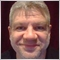# How to calculate correct LOT size for NAS10048

Hi all

I'm about as new here as one can be. Both to trading and to this forum and is my first post here.

Hoping I can get a answer to a question. Google hasn't been able to help.

I want to know how can I calculate my lot size for opening a trade on NAS100.

Is there a known formula? For forex pairs it's quite easy. But I can't find the same info for NAS100.

Example. I want to open a sell order on NAS100. I have \$50000 in my account. I want to risk 10% (\$10000). My stoploss is 200 pips.

How can I calculate the lot size from that?

Thanks!Moderator
32953

Exactly the same way as you would for forex.

But be aware that there may be soe errors due to some brokers using the wrong tick value.

I have known some brokers to give the tick value for NAS100 in USD instead of the account currency.48

Keith Watford:

Exactly the same way as you would for forex.

But be aware that there may be soe errors due to some brokers using the wrong tick value.

I have known some brokers to give the tick value for NAS100 in USD instead of the account currency.

Hi

Doesn't seem to work. Do you have a formula? What is the pip value of NAS100?48

Keith Watford:

Exactly the same way as you would for forex.

But be aware that there may be soe errors due to some brokers using the wrong tick value.

I have known some brokers to give the tick value for NAS100 in USD instead of the account currency.

And what is "tick value"? Never heard that term before  . . .48

Keith Watford:

Exactly the same way as you would for forex.

But be aware that there may be soe errors due to some brokers using the wrong tick value.

I have known some brokers to give the tick value for NAS100 in USD instead of the account currency.

A more real life example.

Lets say I want to open a sell right now.

Current price / pip value: 8877.58

Stoploss: 8888.72

Take Profit: 8710

I want to risk a Half Kelly according to my last month's track record on NAS100. So I want to risk 11% of \$50000

I need to calculate the lot size.

Thanks again.Moderator
32953

Stephan:
And what is "tick value"? Never heard that term before  . . .

Then how do you calculate it for forex?48

Keith Watford:

Then how do you calculate it for forex?

(% of Balance) / SL Pips / 10= lot size (In quoted currency)

So lets say I want to buy GBP/USD.

Current Price: 1.31090

SL: 1.30890

TP: 1.32550

Formula is (11% of \$50000) / 200 / 10 = 2.75 lot size (Correct?)

But to use this formula for NAS100, you need to know the monetary value of 8877.58? and the value of a 0.01 change?48

Stephan:

(% of Balance) / SL Pips / 10= lot size (In quoted currency)

So lets say I want to buy GBP/USD.

Current Price: 1.31090

SL: 1.30890

TP: 1.32550

Formula is (11% of \$50000) / 200 / 10 = 2.75 lot size (Correct?)

But to use this formula for NAS100, you need to know the monetary value of 8877.58? and the value of a 0.01 change?

I must be honest. I'm not sure if that SL pips value is 200 or 20...48

And I'm not sure how the 1:100 leverage comes into it and if it is applicable to this calculation...42817

Stephan:

(% of Balance) / SL Pips / 10= lot size (In quoted currency)

So lets say I want to buy GBP/USD.

Current Price: 1.31090

SL: 1.30890

TP: 1.32550

Formula is (11% of \$50000) / 200 / 10 = 2.75 lot size (Correct?)

But to use this formula for NAS100, you need to know the monetary value of 8877.58? and the value of a 0.01 change?

You don't need to divide by 10, from where this 10 comes from ?

Supposing the account is USD and you are using standard lot (100,000), the correct answer is 27.5 lots.

The correct formula is :

lot = capital to risk / (sl"in ticks"*tickValue).

On Forex, usually 1 tick = 1 point (0.00001 for a 5 digits pair).

The tick value = contract size * tick size * conversion rate (from profit currency to account currency).

tick value is provided by mql code but as Keith noticed, sometimes the value provided by the broker is not correct, you can calculate it yourself.42817

Stephan:
And I'm not sure how the 1:100 leverage comes into it and if it is applicable to this calculation...
The leverage has nothing to do with this calculation.